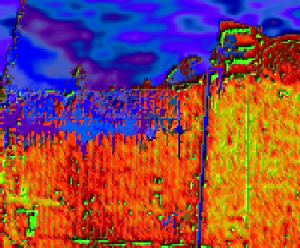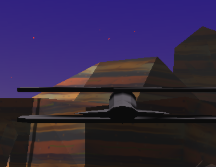﻿ Quantum Mechanics Without Maths ﻿@Template

# 2D quantum mechanics simulations

This is part 2 of the quantum mechanics for programmers article.
Part 1
Let's try a 2D schrodinger equation: $$i \frac{\partial \psi}{\partial t} = -\frac{\partial^2}{\partial x^2}\psi -\frac{\partial^2}{\partial y^2}\psi + \frac{1}{\sqrt{x*x+y*y+\alpha}}\psi.$$

The extra parameter, $\alpha$ isn't a physical thing, but we'll need it to make sure that we don't have a 1/0 value for the potential anywhere.

                // This returns an empty wavefunction of length n.
function wavefunction(n){
var psi = [];
for(var i = 0; i < n; i++){
psi[i]=[];
for(var j = 0; j < n; j++){
psi[i][j] = {real: 0, imag: 0}
}
}
return psi; // for example [{real:0, imag:0}, {real:0, imag:0}, ...]
}
// This takes the starting wavefunction, and returns the wavefunction a short time later.
function timestep(psi)
{
// This is how many units of time we're going to try to step forward.
var dt = 0.02;
psi = timestepV(psi, dt);
psi = timestepT(psi, dt);
return psi;
}
function timestepV(psi, dt)
{

var n = psi.length;
for(var i = 0; i < n; i++)
{
for(var j = 0; j < n; j++){
// This is the x that is in the equation above.
var x = (i-n/2)
var y = (j-n/2)

// This is the potential at this point.
var V = 1.0 / Math.sqrt(x*x + y*y + .01);
var theta = dt * V;
var c = Math.cos(theta);
var s = Math.sin(theta);
var re = psi[i][j].real * c - psi[i][j].imag * s;
var im = psi[i][j].imag * c + psi[i][j].real * s;
psi[i][j].real = re;
psi[i][j].imag = im;
}
}
return psi;
}

function timestepT(psi, dt){
psi = FFT.fft2d(psi);
var n = psi.length;
for(var i = 0; i < n; i++)
{
for(var j=0;j < n; j++)
{
var k = 2 * 3.1415927 * Math.min(i, n-i) / n;
var l = 2 * 3.1415927 * Math.min(j, n-j) / n;

var theta = (k * k + l*l) * dt;
var c = Math.cos(-theta);
var s = Math.sin(-theta);
var re = psi[i][j].real * c - psi[i][j].imag * s;
var im = psi[i][j].imag * c + psi[i][j].real * s;
psi[i][j].real = re;
psi[i][j].imag = im;
}
}
return FFT.fft2d(psi);
}
// This returns the initial state of the simulation.
function init(){
var n = 64;
var psi = wavefunction(n);
for(var i = 0; i < n; i++){
for(var j = 0; j < n; j++){
psi[i][j].real = Math.exp(-((i-20)*(i-20) + (j-20)*(j-20))/(5*5))*4;
psi[i][j].imag = 0;
}
}
return psi;
}



## What next?

If you want to play more with this, to simulate a double slit experiment, or to get it to interact with the mouse, just copy this webpage, and play with the code.

## Context

The technique shown here is good for making simulation videos because it's simple. It's not used all that much in actual research for several reasons:

The task of simulating a 2-D system isn't all that useful. Higher dimensional systems can be simulated too, and there are very many ways of doing this: It's a huge topic. Density functional theory is one example: Effectively, it's just solving very high dimensional quantum mechanics problems. The way it's solved is completely different to the methods discussed here.

For 1-D or 2-D systems, for instance studying 2-atom molecules with known or zero rotational angular momentum, the split-operator method is used, but it's often more accurate / faster to use a chebyshev expansion of the propagator --- my PhD used a chebyshev expansion of the operator to solve Rb2 collision problems.

## Other Articles:## Simulation of flooding in new orleans

A not-very-accurate simulation of the flooding in new orleans.## Experimental Flying Game

Fly around in a plane. Some physics, but mainly just playing with websockets. If you can get a friend to play at the same time, you should be able to shoot each other down.## Atomistic Simulation of Metals

This presents an interactive simulation of atoms making up a nanoscopic particle of metal.